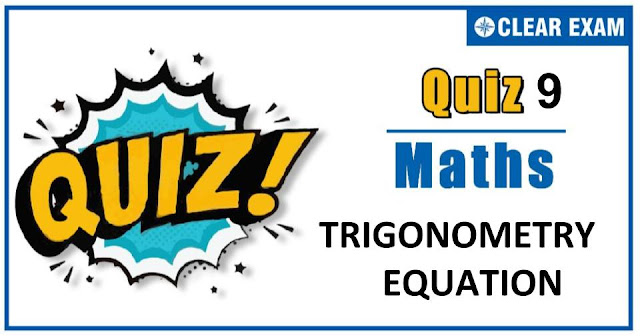## As per analysis for previous years, it has been observed that students preparing for JEE MAINS find Mathematics out of all the sections to be complex to handle and the majority of them are not able to comprehend the reason behind it. This problem arises especially because these aspirants appearing for the examination are more inclined to have a keen interest in Mathematics due to their ENGINEERING background. Furthermore, sections such as Mathematics are dominantly based on theories, laws, numerical in comparison to a section of Engineering which is more of fact-based, Physics, and includes substantial explanations. By using the table given below, you easily and directly access to the topics and respective links of MCQs. Moreover, to make learning smooth and efficient, all the questions come with their supportive solutions to make utilization of time even more productive. Students will be covered for all their studies as the topics are available from basics to even the most advanced.

Q1. The number of solutions of the equation |cos⁡x|=2[x], where [∙] is the greatest integer, is
•  One
•  Two
•  Infinite
•  Nill
Solution
No solution available

Q2.The value of sin⁡10°+sin⁡20°+sin⁡30°+...+sin⁡360° is equal to
•   0
•   1
•  √3
•   2
Solution

Q4. In a ∆ABC,b=2,C=60°,c=√6, then a=
•  √3-1
•  √3
•  √3+1
•  None of these
Solution
Q6. The solution of the equation sin102x=1+cos10x is
•  x=(2n+1) π/2
•  x=nπ
•  x=(2n+1) π/4
•  None of these
Solution
Q8.Consider the following statements :
1. If cosec x=1+cot⁡x,then x=2nπ+3π/4
2. General value of θ satisfying tan^2⁡θ+sec⁡2θ=1 is nπ+π/2
Which of the statements given above is/are correct?
•  Only(1)
•  Only(2)
•  Both (1) and (2)
•  Neither (1) or (2)
Solution
No solution available

Q9.
•   i tanh-1⁡(y)
•  -i tanh-1⁡(y)
•    i tan-1⁡(y)
•   -i tanh-1⁡(y)
Solution
Q10.
•  y=z
•  y+z=a-c
•  y-z=a-c
•  (y-z)=(a-c)2+4b2#### Written by: AUTHORNAME

AUTHORDESCRIPTION## Want to know more

Please fill in the details below:

## Latest NEET Articles\$type=three\$c=3\$author=hide\$comment=hide\$rm=hide\$date=hide\$snippet=hide

Name

ltr
item
BEST NEET COACHING CENTER | BEST IIT JEE COACHING INSTITUTE | BEST NEET, IIT JEE COACHING INSTITUTE: TRIGONOMETRY-EQUATION-QUIZ-9
TRIGONOMETRY-EQUATION-QUIZ-9